# Math in Focus Grade 6 Chapter 9 Lesson 9.2 Answer Key Length of Line Segments

Practice the problems of Math in Focus Grade 6 Workbook Answer Key Chapter 9 Lesson 9.2 Length of Line Segments to score better marks in the exam.

## Math in Focus Grade 6 Course 1 B Chapter 9 Lesson 9.2 Answer Key Length of Line Segments

### Math in Focus Grade 6 Chapter 9 Lesson 9.2 Guided Practice Answer Key

Use graph paper. Plot each pair of points on a coordinate plane. Connect the points to form a line segment and find its length.

Question 1.
E(-6, 0) and D(8, 0)
Point E(-6, 0)
Here, the x-coordinate of E is -6 and the y-coordinate is 0. As the y-coordinate is zero and x coordinate is negative, move 6 units along the negative x-axis and mark it. Thus, the required point(-6,0) is marked.
Point D(8,0)
Here, the x-coordinate of D is 8 and the y-coordinate is 0. As the y-coordinate is zero and x coordinate is positive, move 8 units along the positive x-axis and mark it. Thus, the required point(8,0) is marked.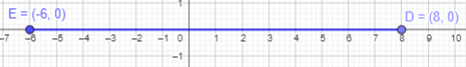After joining the E and D, we will get a line segment of 18 units.

Question 2.
E(-6, 0) and F(-2, 0)
Point E(-6, 0)
Here, the x-coordinate of E is -6 and the y-coordinate is 0. As the y-coordinate is zero and x coordinate is negative, move 6 units along the negative x-axis and mark it. Thus, the required point(-6,0) is marked.
Point F(-2,0)
Here, the x-coordinate of D is -2 and the y-coordinate is 0. As the y-coordinate is zero and x coordinate is negative, move 2 units along the negative x-axis and mark it. Thus, the required point(-2,0) is marked.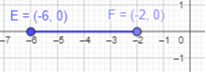After joining the E and F, we will get a line segment of 4 units.

Question 3.
G(-7, 0) and H(1, 0)
Point E(-7, 0)
Here, the x-coordinate of E is -7 and the y-coordinate is 0. As the y-coordinate is zero and x coordinate is negative, move 7 units along the negative x-axis and mark it. Thus, the required point(-7,0) is marked.
Point H(1, 0)
Here, the x-coordinate of H is 1 and the y-coordinate is 0. As the y-coordinate is zero and x coordinate is positive, move 1 units along the positive x-axis and mark it. Thus, the required point(1,0) is marked.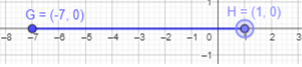After joining the G and H, we will get a line segment of 8 units.

Question 4.
J (0, 5) and K (0, 2)
To plot J(0,5):
Here, the x-coordinate is 0 and the y-coordinate is 5. As the x-coordinate is zero and y coordinate is positive, move 5 units along positive y-axis and mark it. Thus, the required point(0,5) is marked.
To point K (0, 2):
Here, the x-coordinate is 0 and the y-coordinate is 2. As the x-coordinate is zero and y coordinate is positive, move 2 units along positive y-axis and mark it. Thus, the required point(0,2) is marked.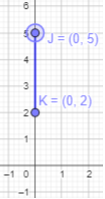After joining the J and K, we will get a line segment of 3 units.

Question 5.
M(0, -6)and N(0, -3)
To plot M(0, -6):
Here, the x-coordinate is 0 and the y-coordinate is -6. As the x-coordinate is zero and y coordinate is negative, move 6 units along negative y-axis and mark it. Thus, the required point (0,-6) is marked.
To point N(0, -3):
Here, the x-coordinate is 0 and the y-coordinate is -3. As the x-coordinate is zero and y coordinate is negative, move 3 units along negative y-axis and mark it. Thus, the required point(0,-3) is marked.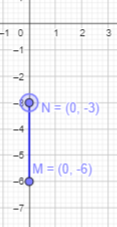After joining the M and N, we will get a line segment of 3 units.

Question 6.
P(0, -3) and Q(0, 5)
To plot P(0, -3):
Here, the x-coordinate is 0 and the y-coordinate is -3. As the x-coordinate is zero and y coordinate is negative, move 3 units along negative y-axis and mark it. Thus, the required point (0,-3) is marked.
To point Q(0, 5):
Here, the x-coordinate is 0 and the y-coordinate is 5. As the x-coordinate is zero and y coordinate is positive, move 5 units along positive y-axis and mark it. Thus, the required point(0,5) is marked.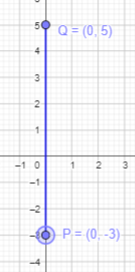After joining the P and Q, we will get a line segment of 8 units.

Use graph paper. Plot each pair of points on a coordinate plane. Connect the points to form a line segment and find its length.

Question 7.
A(1, -2) and B(6, -2)
To plot A(1, -2):
Here, the x-coordinate is 1 and the y-coordinate is -2. Start at the Origin. As the x coordinate is positive, move 1 units to the right along the x-axis and 2 units to the bottom along y -axis.
Thus, the required point (1,-2) is marked.
To point B(6, -2):
Here, the x-coordinate is 6 and the y-coordinate is -2. Start at the Origin. As the x coordinate is positive, move 6 units to the right along the x-axis and 2 units to the bottom along y -axis.
Thus, the required point (6, -2) is marked.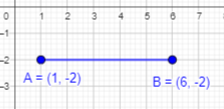After joining the A and B, we will get a line segment of 5 units.

Question 8.
C(-1, 3) and D(5, 3)
Plot C(-1, 3):
Here, the x-coordinate is -1 and the y-coordinate is 3. Start at the Origin. As the x coordinate is negative, move 1 units to the left along the x-axis and 3 units to the above along positive y -axis and mark it.
Thus, the required point (-1, 3) is marked.
Point D(5, 3):
Here, the x-coordinate is 5 and the y-coordinate is 3. Start at the Origin. As the x-coordinate and y coordinate is positive, move 5 unit along positive x-axis and 3 units to the above along positive y -axis and mark it.
Thus, the required point (5, 3) is marked.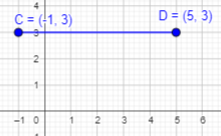After joining the C and D, we will get a line segment of 6 units.

Question 9.
E (-3, 4) and F (1, 4)
Plot E (-3, 4):
Here, the x-coordinate is -3 and the y-coordinate is 4. Start at the Origin. As the x coordinate is negative, move 3 units to the left along the x-axis and 4 units to the above along positive y -axis and mark it.
Thus, the required point (-3, 4) is marked.
Point F (1, 4):
Here, the x-coordinate is 5 and the y-coordinate is 3. Start at the Origin. As the x-coordinate and y coordinate is positive, move 1 unit along positive x-axis and 4 units to the above along positive y -axis and mark it.
Thus, the required point (1, 4) is marked.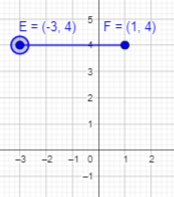After joining the E and F, we will get a line segment of 4 units.

Question 10.
G (-3, 2) and H (-3, 6)
Plot G (-3, 2):
Here, the x-coordinate is -3 and the y-coordinate is 2. Start at the Origin. As the x coordinate is negative, move 3 units to the left along the x-axis and 2 units to the above along positive y -axis and then mark it.
Thus, the required point (-3, 2) is marked.
Point H (-3, 6):
Here, the x-coordinate is -3 and the y-coordinate is 6. Start at the Origin. As the x-coordinate is negative, move 3 units to the left along the x-axis and 6 units along positive y-axis and then mark it.
Thus, the required point (-3, 6) is marked.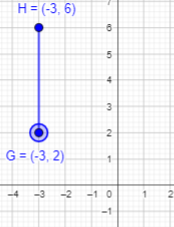After joining the G and H, we will get a line segment of 4 units.

Question 11.
J(-1, -6) and K(-1, 4)
Plot J(-1, -6):
Here, the x-coordinate is -1 and the y-coordinate is -6. Start at the Origin. As the x coordinate is negative, move 1 unit to the left along the x-axis and 6 units along negative y -axis and then mark it.
Thus, the required point (-1, -6) is marked.
Point K(-1, 4):
Here, the x-coordinate is -1 and the y-coordinate is 4. Start at the Origin. As the x-coordinate is negative, move 1 units to the left along the x-axis and 4 units along positive y-axis and then mark it.
Thus, the required point (-1, 4) is marked.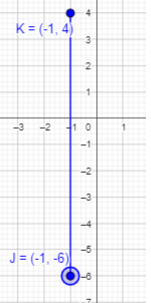After joining the J and K, we will get a line segment of 10 units.

Question 12.
L(5, 6) and M(5, 1)
Point L(5, 6):
Here, the x-coordinate is 5 and the y-coordinate is 6. Start at the Origin. As the x-coordinate and y coordinate is positive, move 5 units along positive x-axis and 6 units to the above along positive y -axis and mark it.
Thus, the required point (5, 6) is marked.
Point M(5, 1):
Here, the x-coordinate is 5 and the y-coordinate is 1. Start at the Origin. As the x-coordinate and y coordinate is positive, move 5 units along positive x-axis and 1 unit to the above along positive y -axis and mark it.
Thus, the required point (5, 1) is marked.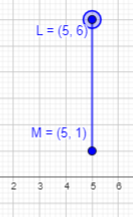After joining the L and M, we will get a line segment of 5 units.

In the diagram, triangle ABC represents a plot of land. The side length of each grid square ¡s 5 meters. Use the diagram to answer questions 13 to 15.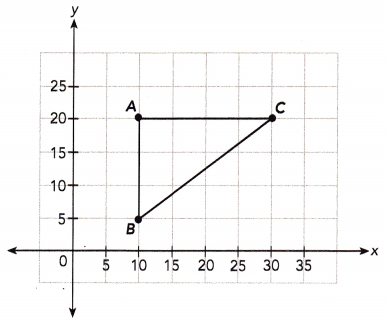Question 13.
Give the coordinates of points A, B, and C.
Point A:
The first coordinate represents distance along x axis which is 10 units and the second coordinate represents distance along y axis which is 20 units.
So, the point A will be (10,20).
Point B:
The first coordinate represents distance along x axis which is 10 units and the second coordinate represents distance along y axis which is 5 units.
So, the point B will be (10,5).
Point C:
The first coordinate represents distance along x axis which is 30 units and the second coordinate represents distance along y axis which is 20 units.
So, the point C will be (30,20).

Question 14.
Mr. Manning wants to build a fence around the plot of land. If BC is 25 meters, how many meters of fencing does he need?
AB =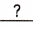=m
AC =Perimeter of triangle ABC = AB + BC + AC
=++=m
Mr. Manning needsmeters of fencing.
Here, 1 grid square represents 5 meters. From the figure,
AB equals 3 grid square so it will measure 3×5=15m
AC equals 4 grid square so it will measure 4×5=20m
Given BC equals 25m.
Perimeter of triangle ABC = AB + BC + AC
= 15+25+20
= 60 m

Question 15.
A pole is located at point D on the plot of land at a distance of 10 meters from $$\overline{A B}$$ and 5 meters from $$\overline{A C}$$. Give the coordinates of point D.
1 grid square represents 5 meters.
10 m =÷ 5
=grid squares
Given that point D is located a distance of 10 meters from $$\overline{A B}$$ and 5 meters from $$\overline{A C}$$.
10 m = 10 ÷ 5
= 2 grid squares.

For point D to be on the plot of land, the x-coordinate has to begrid squares to the right of $$\overline{\mathrm{AB}}$$. So, point D is+=grid squares to the right of the y-axis. The x-coordinate of point D is×=.
For point D to be on the plot of land, the x-coordinate has to be 2 grid squares to the right of
$$\overline{\mathrm{AB}}$$. So, point D is 2+2 = 4 grid squares to the right of the y-axis.
The x-coordinate of point D is 4×5  = 20.

For point D to be on the plot of land, the y-coordinate has to begrid square below $$\overline{\mathrm{AC}}$$. So, point D is=grid squares above the x-axis. The y-coordinate of point D is×=.
The coordinates of D are (,)
For point D to be on the plot of land, the y-coordinate has to be 1 grid square below $$\overline{\mathrm{AC}}$$. So, point D is 4-1 = 3 grid squares above the x-axis. The y-coordinate of point D is 3×5  = 15.
The coordinates of D are (20,15)

In the diagram, rectangle PQRS represents a parking lot of a supermarket. The side length of each grid square is 4 meters. Use the diagram to answer questions 16 to 18.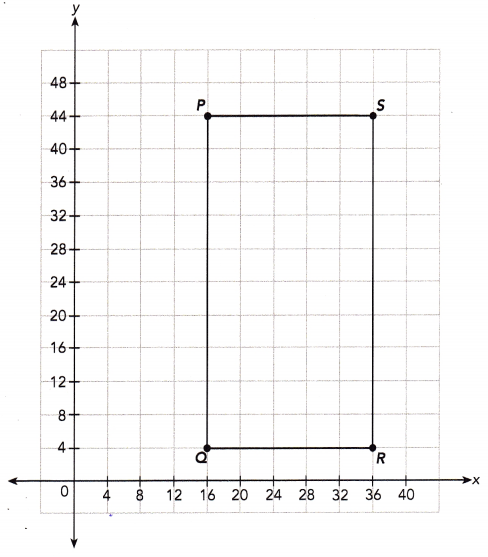Question 16.
Give the coordinates of points P, Q, R, and S.
Point P:
The first coordinate represents distance along x axis which is 16 units and the second coordinate represents distance along y axis which is 44 units.
So, the point P will be (16,44).
Point Q:
The first coordinate represents distance along x axis which is 16 units and the second coordinate represents distance along y axis which is 4 units.
So, the point Q will be (16,4).
Point R:
The first coordinate represents distance along x axis which is 36 units and the second coordinate represents distance along y axis which is 4 units.
So, the point QRwill be (36,4).
Point S:
The first coordinate represents distance along x axis which is 36 units and the second coordinate represents distance along y axis which is 44 units.
So, the point S will be (36,44).

Question 17.
The manager of the supermarket wants to build a concrete wall around the parking lot. What is the perimeter of the wall?
Here, 1 grid square represents 4 meters.
Length of PQ = 44-4 = 40 m
Length of QR = 36-16 = 20m
Since PQRS is a rectangle, opposite lengths will be equal.
Perimeter of wall will be = 40+20+40+20 = 120m

Question 18.
The entrance of the supermarket is at point T. It lies on $$\overline{P Q}$$, and point T is 8 meters from point P. Give the coordinates of point T.
Point T lies on $$\overline{P Q}$$, and it is 8 meters from point P.
Thus, the entrance will be on PQ.
8 m means 8÷4=2 square grid
Move 2 square grid below point P on $$\overline{P Q}$$
Thus, point T will be (16,36)

### Math in Focus Course 1B Practice 9.2 Answer Key

Use graph paper. Plot each pair of points on a coordinate plane. Connect the points to form a line segment and find its length.

Question 1.
A(5, 0) and B(8, 0)
Point A(5, 0)
Here, the x-coordinate is 5 and the y-coordinate is 0. As the y-coordinate is zero and x coordinate is positive, move 5 units along the positive x-axis and mark it. Thus, the required point(5,0) is marked.
Point B(8,0)
Here, the x-coordinate is 8 and the y-coordinate is 0. As the y-coordinate is zero and x coordinate is positive, move 8 units along the positive x-axis and mark it. Thus, the required point(8,0) is marked.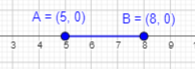After joining the A and B, a line segment of 3 units is formed.

Question 2.
C(-3, 4)and D(3, 4)
Plot C(-3, 4):
Here, the x-coordinate is -3 and the y-coordinate is 4. Start at the Origin. As the x coordinate is negative, move 3 units along the negative x-axis and 4 units to the above along positive y -axis and mark it.
Thus, the required point (-3, 4) is marked.
Plot D(3, 4):
Here, the x-coordinate is 3 and the y-coordinate is 4. Start at the Origin. As the x coordinate is positive, move 3 units along the positive x-axis and 4 units to the above along positive y -axis and mark it.
Thus, the required point (3, 4) is marked.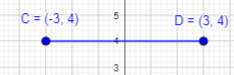After joining the C and D, a line segment of 6 units is formed.

Question 3.
E (-5, -2) and F (8, -2)
Plot point E(-5,-2)
Here, the x-coordinate is -5 and the y-coordinate is -2. Start at the Origin. As the x coordinate is negative, move 5 units along the negative x-axis and 2 units along negative y -axis and mark it.
Thus, the required point (-5, -2) is marked.
Plot point F(8, -2)
Here, the x-coordinate is 8 and the y-coordinate is -2. Start at the Origin. As the x coordinate is positive, move 8 units along the positive x-axis and 2 units along negative y -axis and mark it.
Thus, the required point (8, -2) is marked.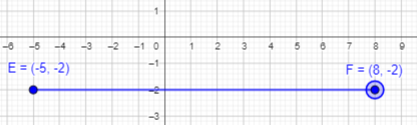After joining the E and F, a line segment of 13 units is formed.

Question 4.
G(0, -5) and H(0, 2)
Plot point G(0, -5)
Here, the x-coordinate is 0 and the y-coordinate is -5. As the x-coordinate is zero and y coordinate is negative, move 5 units along negative y-axis and mark it. Thus, the required point(0,-5) is marked.
Plot point H(0, 2)
Here, the x-coordinate is 0 and the y-coordinate is 2. As the x-coordinate is zero and y coordinate is positive, move 2 units along positive y-axis and mark it. Thus, the required point(0,2) is marked.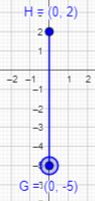After joining the G and H, a line segment of 7 units is formed.

Question 5.
J(-5, -3) and K(-5, -8)
Plot point J(-5, -3)
Here, the x-coordinate is -5 and the y-coordinate is -3. Start at the Origin. As the x coordinate is negative, move 5 units along the negative x-axis and 3 units along negative y -axis and mark it.
Thus, the required point (-5, -3) is marked.
Plot point K(-5,-8):
Here, the x-coordinate is -5 and the y-coordinate is -8. Start at the Origin. As the x coordinate is negative, move 5 units along the negative x-axis and 8 units along negative y -axis and mark it.
Thus, the required point (-5, -8) is marked.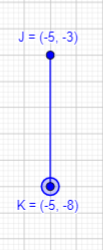After joining the J and K, a line segment of 5 units is formed.

Question 6.
M(1, 7) and N(1, -8)
Plot point M(1, 7)
Here, the x-coordinate is 1 and the y-coordinate is 7. Start at the Origin. As the x coordinate is positive, move 1 unit along the positive x-axis and 7 units along positive y -axis and mark it.
Thus, the required point (1, 7) is marked.
Plot point N(1, -8)
Here, the x-coordinate is 1 and the y-coordinate is -8. Start at the Origin. As the x coordinate is positive, move 1 unit along the positive x-axis and 8 units along negative y -axis and mark it.
Thus, the required point (1, -8) is marked.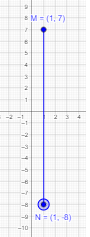After joining the M and N, a line segment of 15 units is formed.

Use graph paper. Find the coordinates.

Question 7.
Rectangle PQRS is plotted on a coordinate plane. The coordinates of P are (-1, -3) and the coordinates of Q are (-1, 2). Each unit on the coordinate plane represents 1 centimeter, and the perimeter of rectangle PQRS is 20 centimeters. Find the coordinates of points R and S given these conditions:
a) Points R and S are to the left of points P and Q.
Given that PQRS is a rectangle. The coordinates of P are (-1, -3) and the coordinates of Q are (-1, 2).
The perimeter of rectangle PQRS is 20 centimeters.
Length of PQ is 5 units or 5 cm which is equal to length of RS. Length of RQ is equal to length of SP.
Perimeter of rectangle = Sum of four sides
20 = PQ+QR+RS+SP
20 = 5+QR+5+SP
20 = 5+SP+5+SP
20 = 10+2×SP
20-10 = 10+2×SP-10
10 = 2×SP
10÷2 = (2×SP)÷2
5 = SP
Length of SP equals to 5 units or 5 cm.
Start from point P and move 5 square grids towards left and point R. Similarly, point R from Q and then join the points to form the rectangle.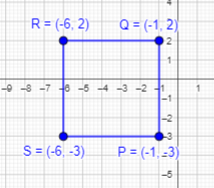b) Points R and S are to the right of points P and Q. The coordinates of P are (-1, -3) and the coordinates of Q are (-1, 2).
We know the measurements of the PQRS, where length of all sides are equal.
Start from point P and move 5 square grids towards right and point R. Similarly, point R1 from Q and then join the points to form the rectangle.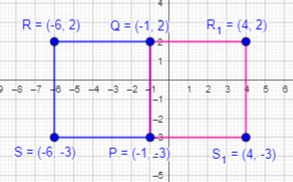Question 8.
Rectangle ABCD is plotted on a coordinate plane. The coordinates of A are (2, 3) and the coordinates of B are (-2, 3). Each unit on the coordinate plane represents 3 centimeters, and the perimeter of rectangle ABCD is 48 centimeters. Find the coordinates of points C and D given these conditions:
a) Points C and D are below points A and B.
Given that ABCD is a rectangle. The coordinates of A are (2, 3) and the coordinates of B are (-2, 3).
The perimeter of rectangle ABCD is 48 centimeters.
Length of AB is 4 units or 4×3 = 12cm which is equal to length of CD. Length of AC is equal to length of BD.
Perimeter of rectangle = Sum of four sides
48 = AB+BD+DC+CA
48 = 12+3BD+12+3BD (BD will be equal to 3BD as length of each unit on the coordinate plane represents 3 cm)
48 = 24+2×6×BD
48-24 = 24+12×BD-24
24 = 12×BD
24÷12 = (12×BD)÷12
2= BD
Length of BD equals to 2 units or 2×3=6 cm.
Start from point A and move 2 square grids below and point C. Similarly, point D from B and then join the points to form the rectangle.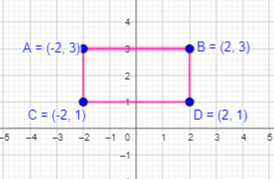b) Points C and D are above points A and B.
We know the measurements of the ABCD, where length of opposite sides are equal.
Start from point A and move 2 square grids upwards and point C1. Similarly, point D1 from B and then join the points to form the rectangle.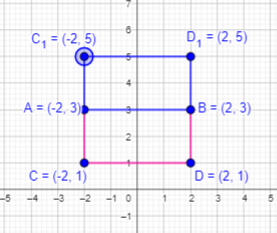Question 9.
Rectangle PQRS is plotted on a coordinate plane. The coordinates of P are (-1, 4) and the coordinates of Q are (-1, -4). Each unit on the coordinate plane represents 1 centimeter, and the area of rectangle PQRS is 64 square centimeters. Find the coordinates of points R and S given these conditions:

a) Points R and S are to the left of points P and Q.
Given that PQRS is a rectangle. The coordinates of P are (-1, 4) and the coordinates of Q are (-1, -4).
The area of rectangle PQRS is 64 sq.cm
Length of PQ is 8 units or 8 cm which is equal to length of RS. Length of QS is equal to length of RP.
Area of rectangle = length×width
64 = PQ×QR
64 = 8×QR
64÷8 = (8×QR)÷8
8 = QR
Length of QR equals to 8 units or 8 cm.
Start from point P and move 8 square grids to the left and mark point R. Similarly, mark point S from Q and then join the points to form the rectangle.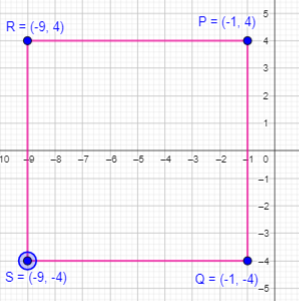b) Points R and S are to the right of points P and Q.
We know the measurements of the PQRS, where length of all sides are equal.
Start from point P and move 8 square grids towards right and point it as R. Similarly, point S from Q and then join the points to form the rectangle.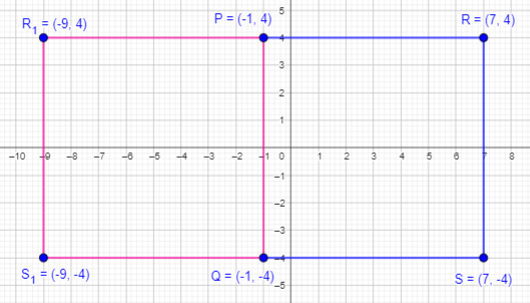In the diagram, rectangle ABCD represents a shopping plaza. The side length of each grid square Is 10 meters. Use the diagram to answer questions 10 to 14.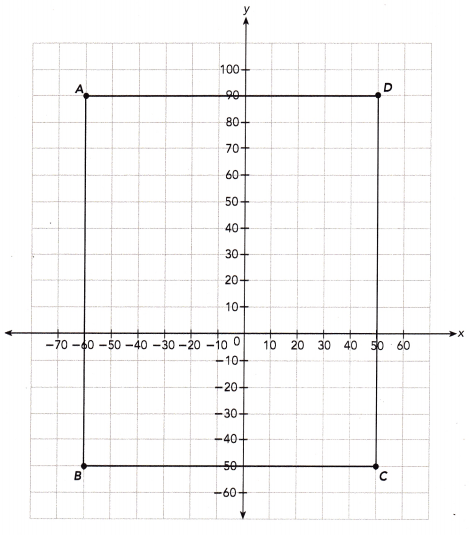Question 10.
Give the coordinates of points A, B, C, and D.
Point A:
The first coordinate represents distance along x axis which is 60 units and the second coordinate represents distance along y axis which is 90 units.
So, the point A will be (-60,90).
Point B:
The first coordinate represents distance along x axis which is 60 units and the second coordinate represents distance along y axis which is 50 units.
So, the point A will be (-60,50).
Point C:
The first coordinate represents distance along x axis which is 50 units and the second coordinate represents distance along y axis which is 50 units.
So, the point A will be (50,-50).
Point D:
The first coordinate represents distance along x axis which is 50 units and the second coordinate represents distance along y axis which is 90 units.
So, the point A will be (50,90).

Question 11.
Write down the shortest distance of points A, B, C, and D from the y-axis.
The shortest distance of points A from the y-axis is 60 units.
The shortest distance of points B from the y-axis is 60 units.
The shortest distance of points C from the y-axis is 50 units.
The shortest distance of points D from the y-axis is 50 units.

Question 12.
Write down the shortest distance of points A, B, C, and D from the x-axis.
The shortest distance of points A from the x-axis is 90 units.
The shortest distance of points B from the x-axis is 50 units.
The shortest distance of points C from the x-axis is 50 units.
The shortest distance of points D from the x-axis is 90 units.

Question 13.
Find the area and perimeter of the shopping plaza.
The side length of each grid square Is 10 meters.
Length of AB is 140 square grid, 140×10=1400 m
Length of BC is 110 square grid, 110×10=1100 m
Thus, the length of the shopping plaza is 1400m and width is 1100m
Area of the shopping plaza = length × width
= 1400×1100
= 1540000 sq.m
Perimeter of the shopping plaza = 2×(length+width)
= 2×(1400+1100)
= 2×2500
= 5000 m

Question 14.
A man at the shopping plaza is standing 50 meters from $$\overline{\mathrm{AD}}$$, and 40 meters from $$\overline{\mathrm{DC}}$$.
a) Find the coordinates of the point representing the man’s location.
Given that:
A man at the shopping plaza is standing 50 meters from $$\overline{\mathrm{AD}}$$
AD – 50 = 90-50 = 40
and 40 meters from $$\overline{\mathrm{DC}}$$.
DC – 40 = 50-40 = 10

b) Find the shortest distance in meters from the man’s location to the side $$\overline{\mathrm{BC}}$$.
Count the square grids from the man’s location to the side BC.
There are 9 square grids, 9×10 = 90m
Thus, 90m is the shortest distance in meters from the man’s location to the side BC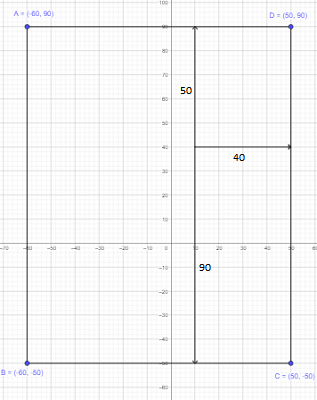In the diagram, triangle PQR represents a triangular garden. The side length of each grid square Is 5 meters. Use the diagram to answer questions 15 to 19.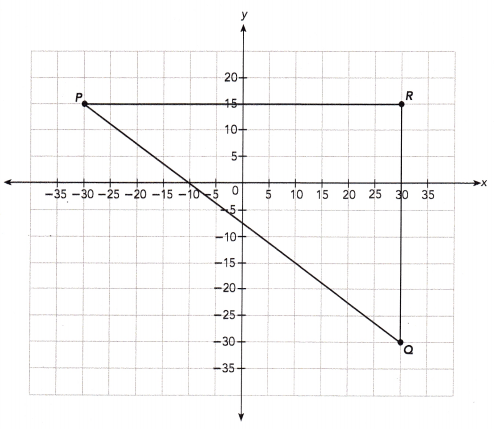Question 15.
Use graph paper. A rectangular region ABCR in the garden is to be fenced in. Point A lies on $$\overline{P R}$$, and is 35 meters away from point P. Point C lies below $$\overline{P R}$$, and is 20 meters away from point R. Plot and label points A, B, and C on the coordinate plane. Write the coordinates of points A, B, and C.
A rectangular region ABCR in the garden is to be fenced in. Point A lies on $$\overline{P R}$$, and is 35 meters away from point P.
The side length of each grid square Is 5 meters.
Since point A is 35m away from P, 35÷5=7 square grids.
Move 7 units away from point P and mark it as A.
Thus, the point A will be (5,15)

Point C lies below $$\overline{P R}$$, and is 20 meters away from point R.
Since point C is 20m away from point R, 20÷5=4 square grids.
Move 4 units to the down from point R and mark it as C.
Thus, the point C will be (30, -5)

Since ABCR is a rectangular region, the opposite sides are equal.
Start from point C and move 5 units towards left, and mark the point as B.
Thus, the point B will be (5,-5)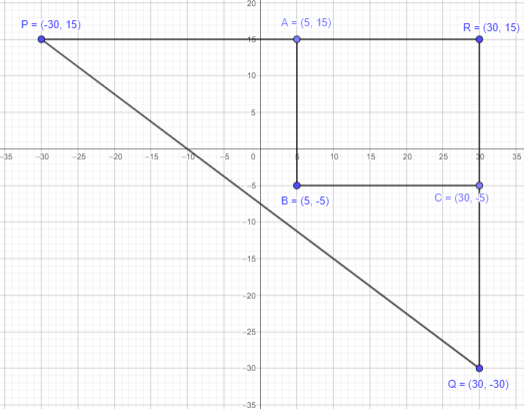If PQ is 75 meters, what is the perimeter of the triangular garden in meters?
Given that PQ is 75 m.
Length of PR = 12 square grids.
Here, one square grid equals 5m. So, 12 square grids = 12×5 = 60m
Length of RQ = 9 square grids.
So, 9 square grids = 9×5 = 45m
Perimeter of the triangular garden = 75+60+45 = 180m

Question 17.
Find the area of the enclosed region ABCR in square meters.
Length of AR = 5 square grids, 5×5=25m
Width of AB = 4 square grids, 4×5=20m
Area of rectangle = length×width
=25×20
=500
Area of the enclosed region ABCR will be 500 sq.m

Question 18.
Find the perimeter of the enclosed region ABCR in meters.
Length of AR = 25m
Width of AB = 20m
Perimeter = AR+RC+CB+BA
= 25+20+25+20
= 90 m

Question 19.
If PQ is 75 meters, what is the perimeter of the garden that is not enclosed?
Given PQ is 75m.
Length of AP = 7 square grids,7×5=35 m
Length of AB = 4 square grids,4×5=20 m
Length of BC = 5 square grids,5×5=25 m
Length of CQ = 5 square grids,5×5=25 m
Perimeter of the garden that is not enclosed = 75+35+20+25+25 = 180 m

The diagram shows the outline of a park. The side length of each grid square is 10 meters. Use the diagram to answer questions 20 to 22.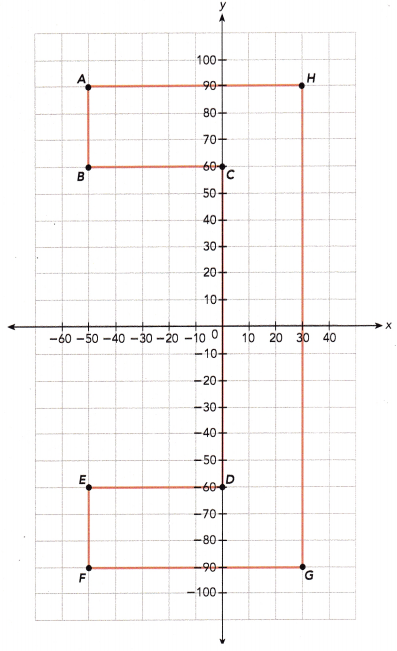Question 20.
Find the area of the park in square meters.
Let us assume AHGF as a rectangle, AH be the length and HG be the width.
Length of AH is 80m and length of HG is 180m.
Area of rectangle = length×width
= 80×180
= 14400 sq.m
Area of AHGFA will be 14400 sq.m
Area of rectangle BCDE:
Length of BC = 50m
Length of CD = 120m
Area of BCDE will be 50×120 = 6000 sq.m
Area of the park can be calculated by subtracting the area of rectangle BCDE from the rectangle AHGF
= 14400-6000
= 8400 sq.m

Question 21.
Brandon starts at point A and walks all the way around the perimeter of the park. If he walks at 1.5 meters per second, about how many seconds pass before he returns to point A? Round your answer to the nearest second.
The side length of each grid square Is 10 m.
Length of HA: 8 square grids = 8×10 = 80 m
Length of AB: 3 square grids = 3×10 = 30 m
Length of BC: 5 square grids = 5×10 = 50 m
Length of CD: 12 square grids = 12×10 = 120 m
Length of DE: 5 square grids = 5×10 = 50 m
Length of EF: 3 square grids = 3×10 = 30 m
Length of FG: 8 square grids = 8×10 = 80 m
Length of GH: 8 square grids = 18×10 = 180 m
Perimeter of the park will be 80+30+50+120+50+30+80+180 = 620m
He walks at 1.5 meters per second.
620÷1.5 = 413.3
Round off for 413.3 equals to 413 sec

Question 22.
A picnic table in the park is 20 meters from $$\overline{B C}$$, and is closer to point B than it is to point C. Write down two possible pairs of coordinates for the location of the picnic table.
Given that a picnic table in the park is 20 m from $$\overline{B C}$$, and is closer to point B than it is to point C.